These illustrations are suggested in the endnotes of Political Games. This document sets up the code and implements all these analyses. You can replicate–or modify–any figure using the code fragments below.

# 1 Getting Going

First open R or Rstudio and install the hop package from github:

``devtools::install_github("macartan/hop")``

``library(hop)``

# 2 Implement

Some normal form games: PD (#1), with and without equilibrium marked

``````payoffs <-  matrix(c(2,3,0,1),2)
gt_bimatrix(payoffs, labels1=c("C","D"))``````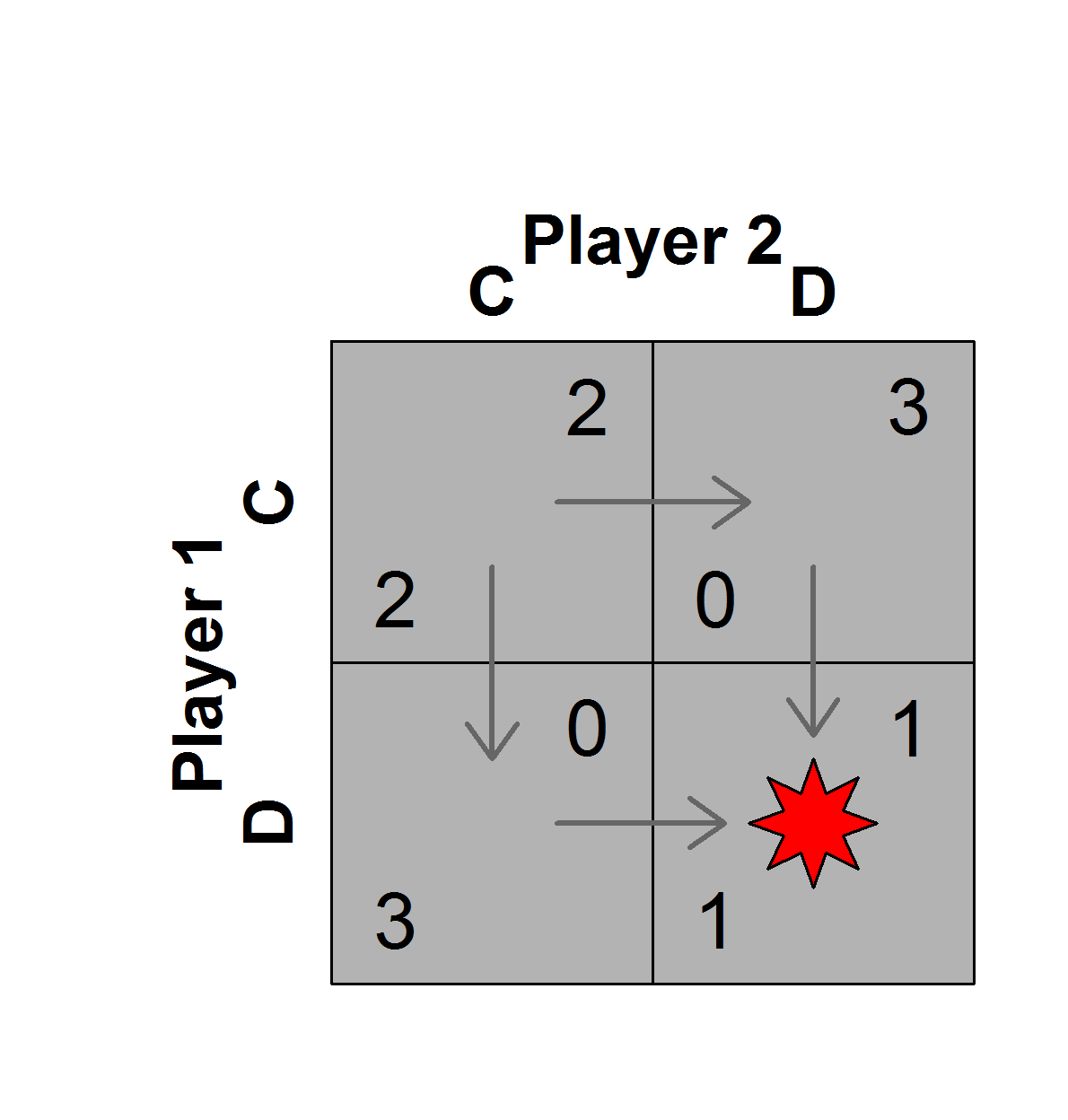``gt_bimatrix(payoffs, labels1=c("C","D"), nash=F, arrow1=F)``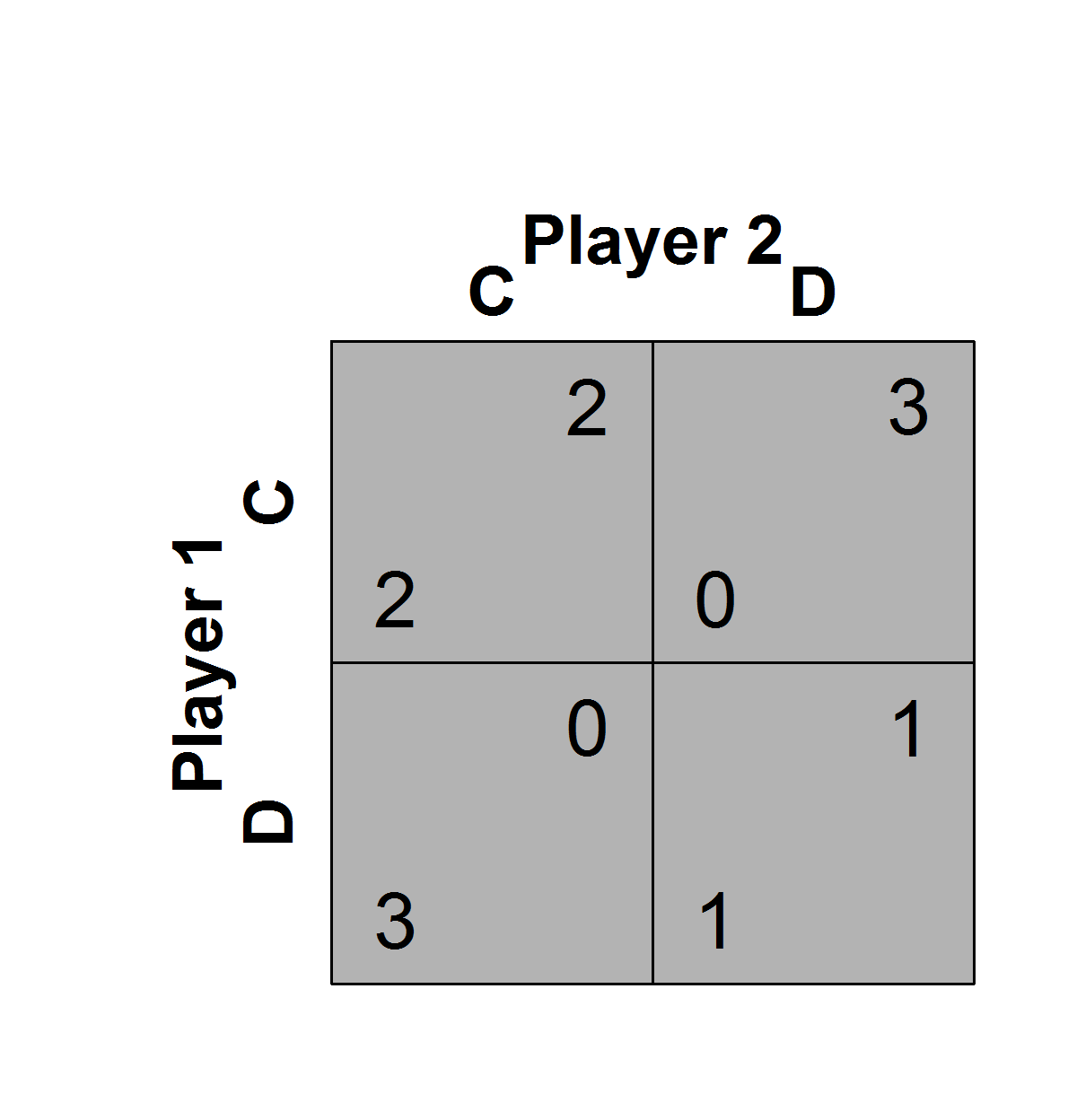Chicken (#2): Pure strategy equilibriums marked

``````payoffs <- matrix(c(2,3,1,0),2)
gt_bimatrix(payoffs, labels1=c("C","D"))``````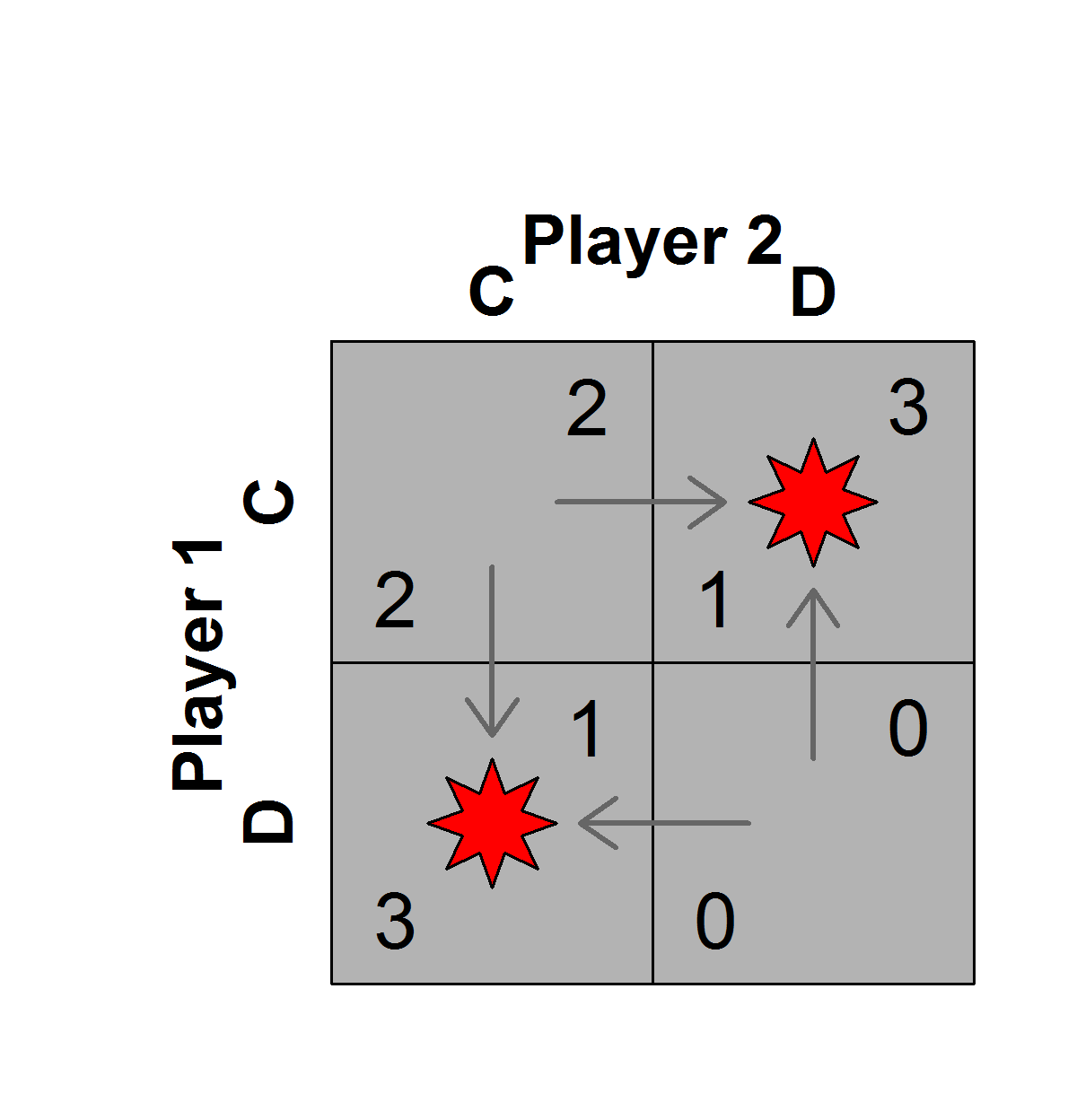Assurance (#3): Pure strategy equilibriums marked

``````payoffs <- matrix(c(3,2,0,1),2)
gt_bimatrix(payoffs, labels1=c("C","D"))``````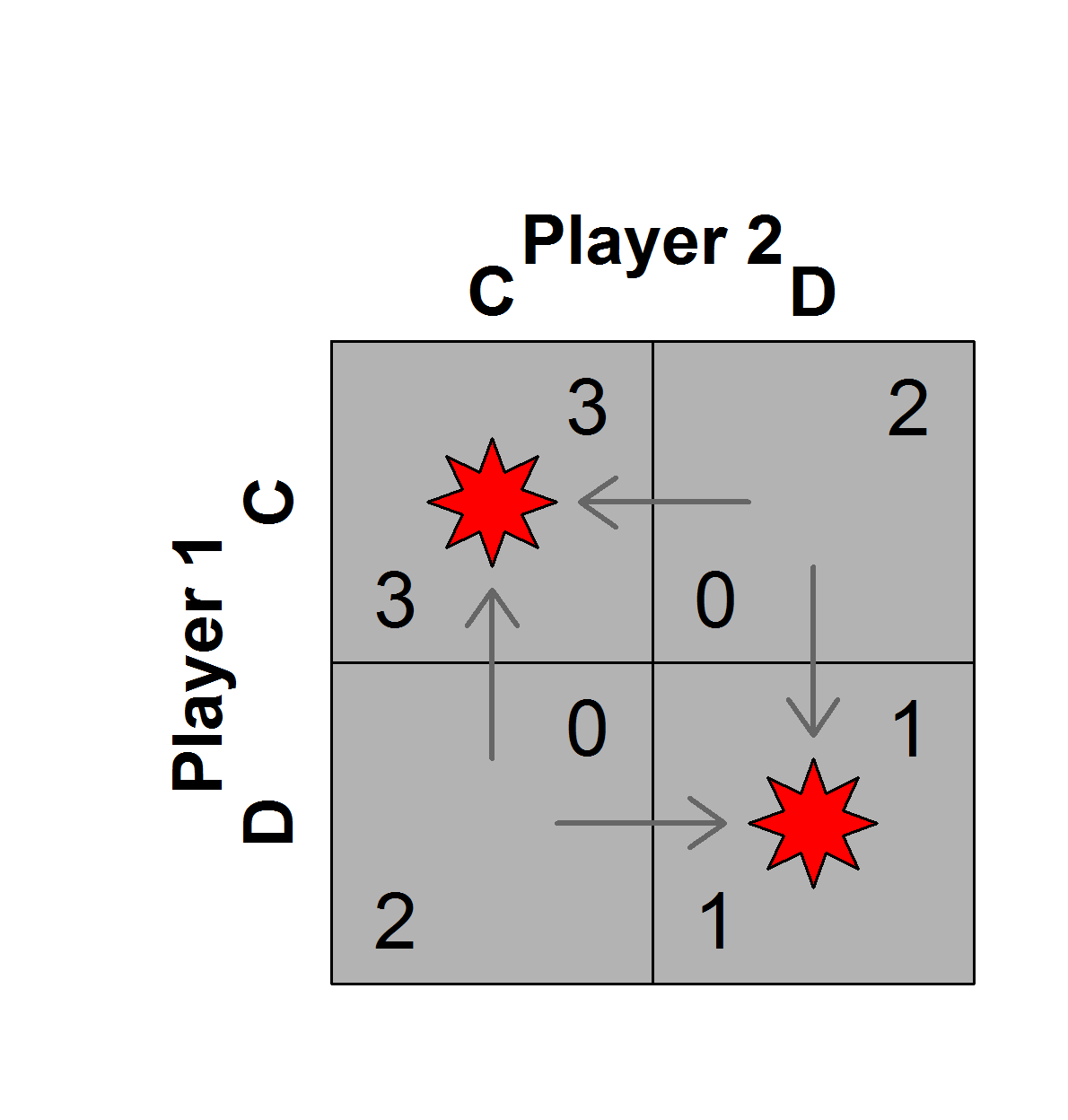Illustrating the folk theorems (#4) for the Prisoner’s Dilemma:

``````payoffs <- matrix(c(2,3,0,1),2)
gt_folk(payoffs)``````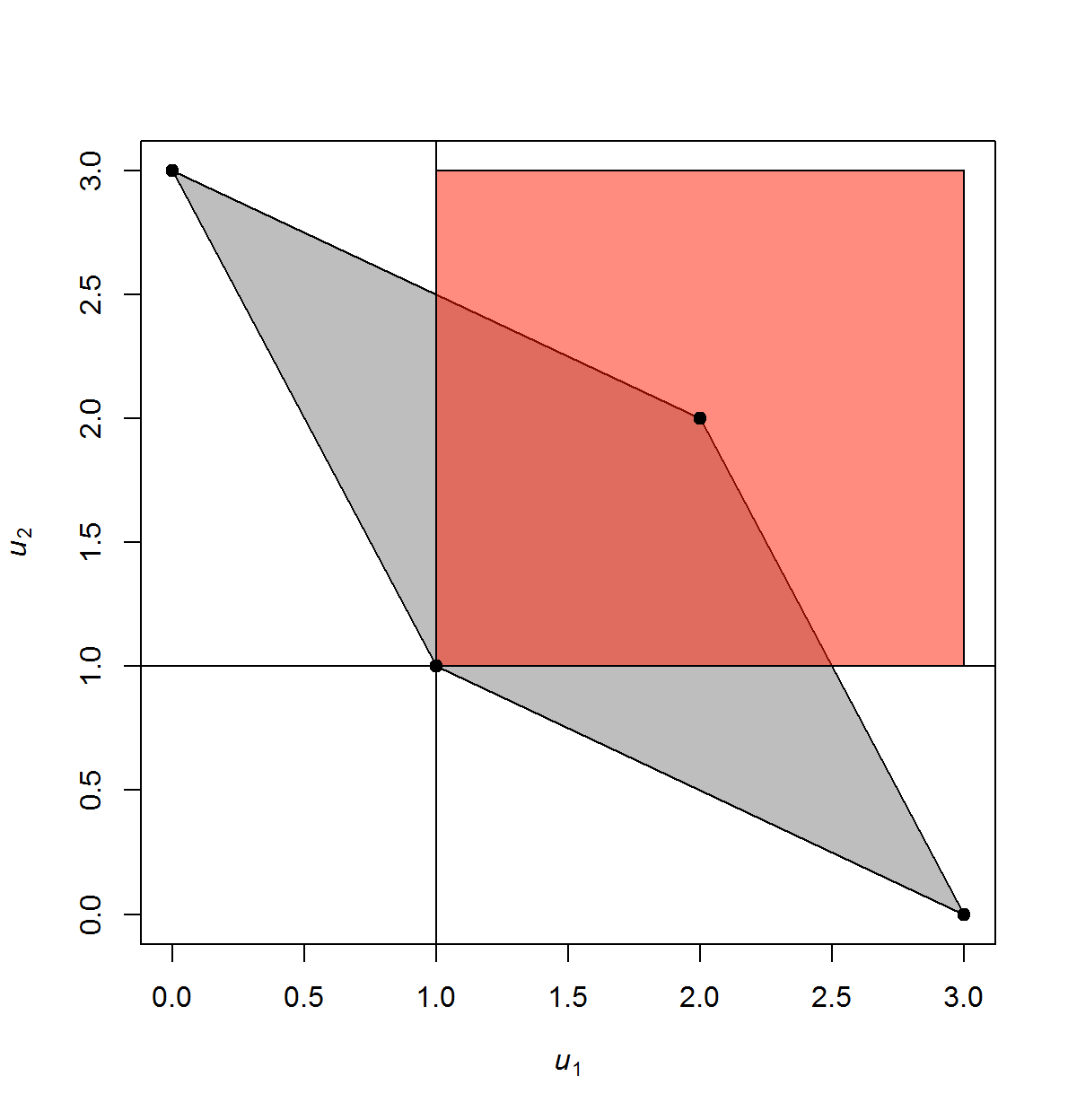…and for the Assurance Game

``````payoffs <- matrix(c(3,2,0,1),2)
gt_folk(payoffs)``````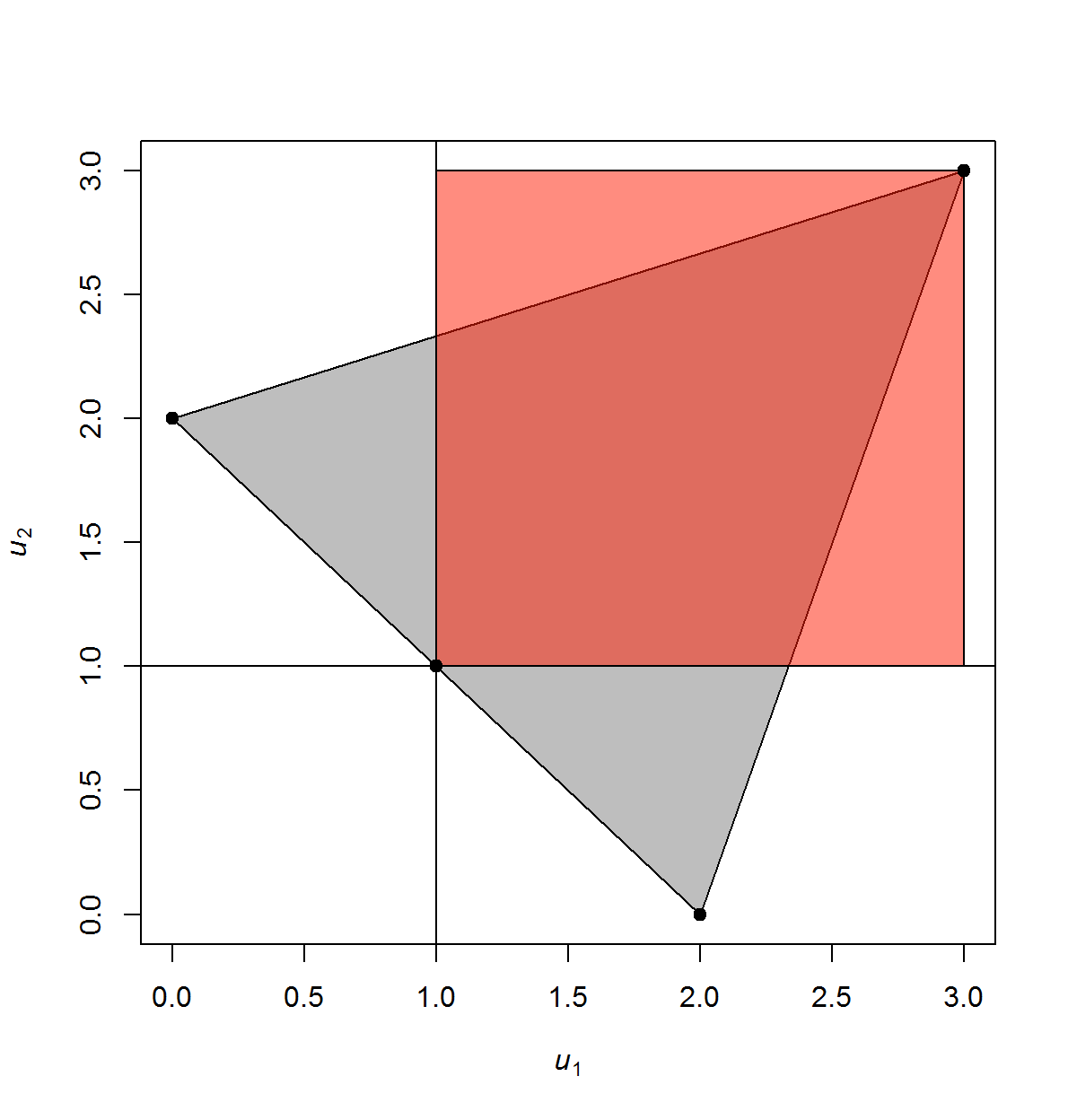Condorcet Jury (#10): Gives an illustration of the law of large numbers

``gt_jury(n_voters=7, probability_correct=.6)``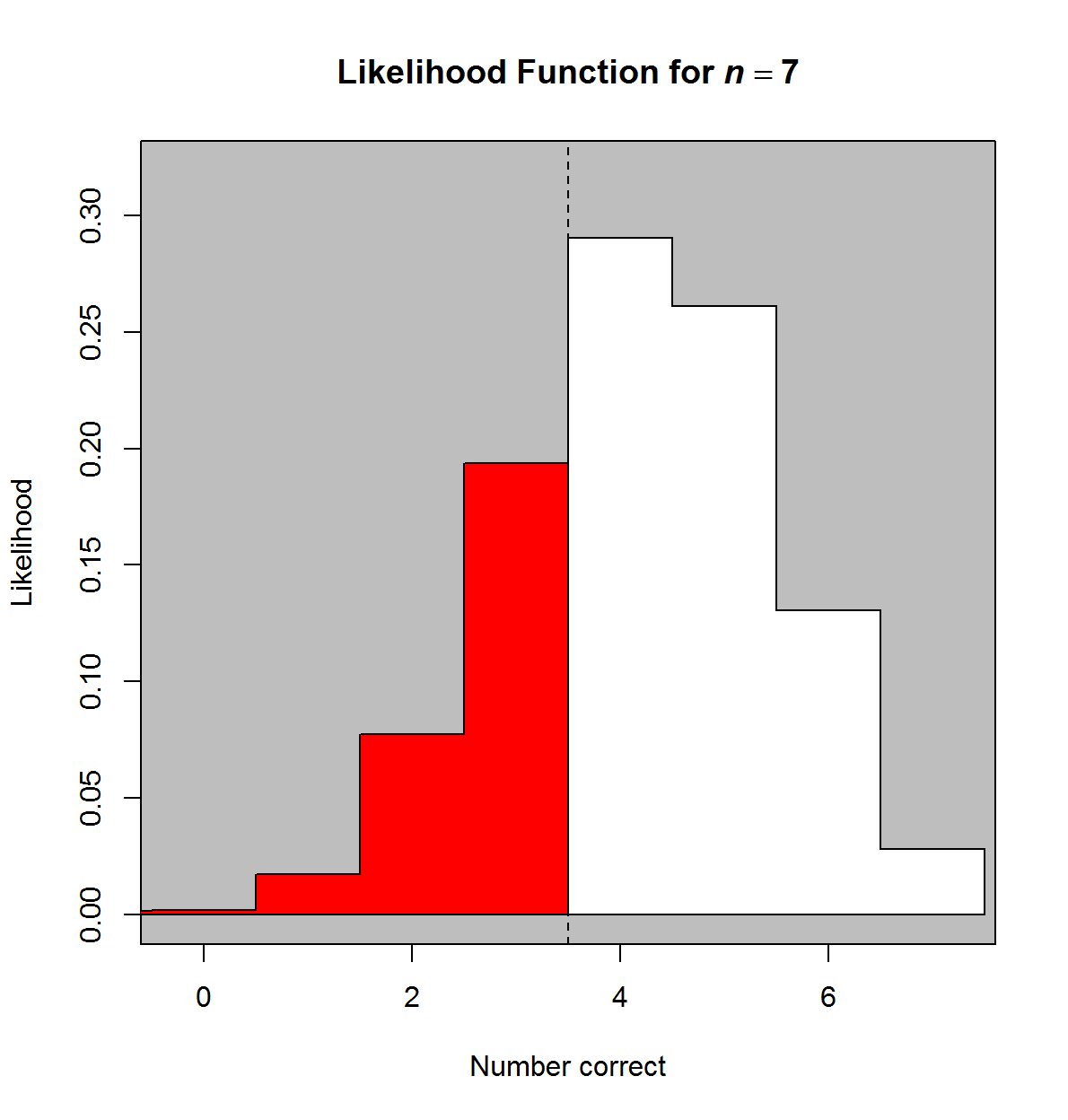Power indices (#13): Shows mapping between raw votes and power for two seemingly similar committees

``gt_plot_banzhaf(weights = c(1, 1, 3,7,9,9), q_rule = .5)``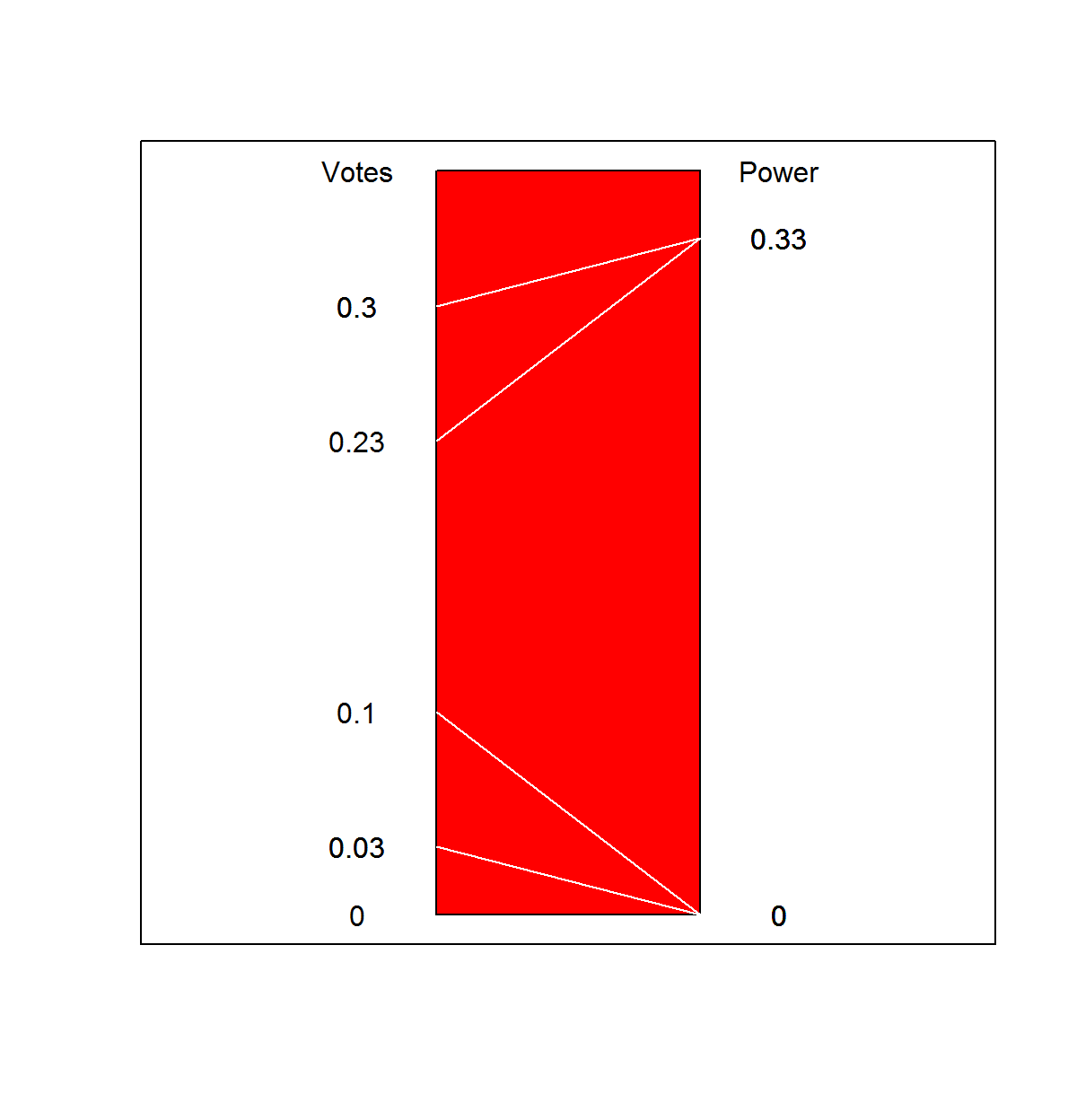``gt_plot_banzhaf(weights = c(1, 1, 3,7,9,10), q_rule = .6)``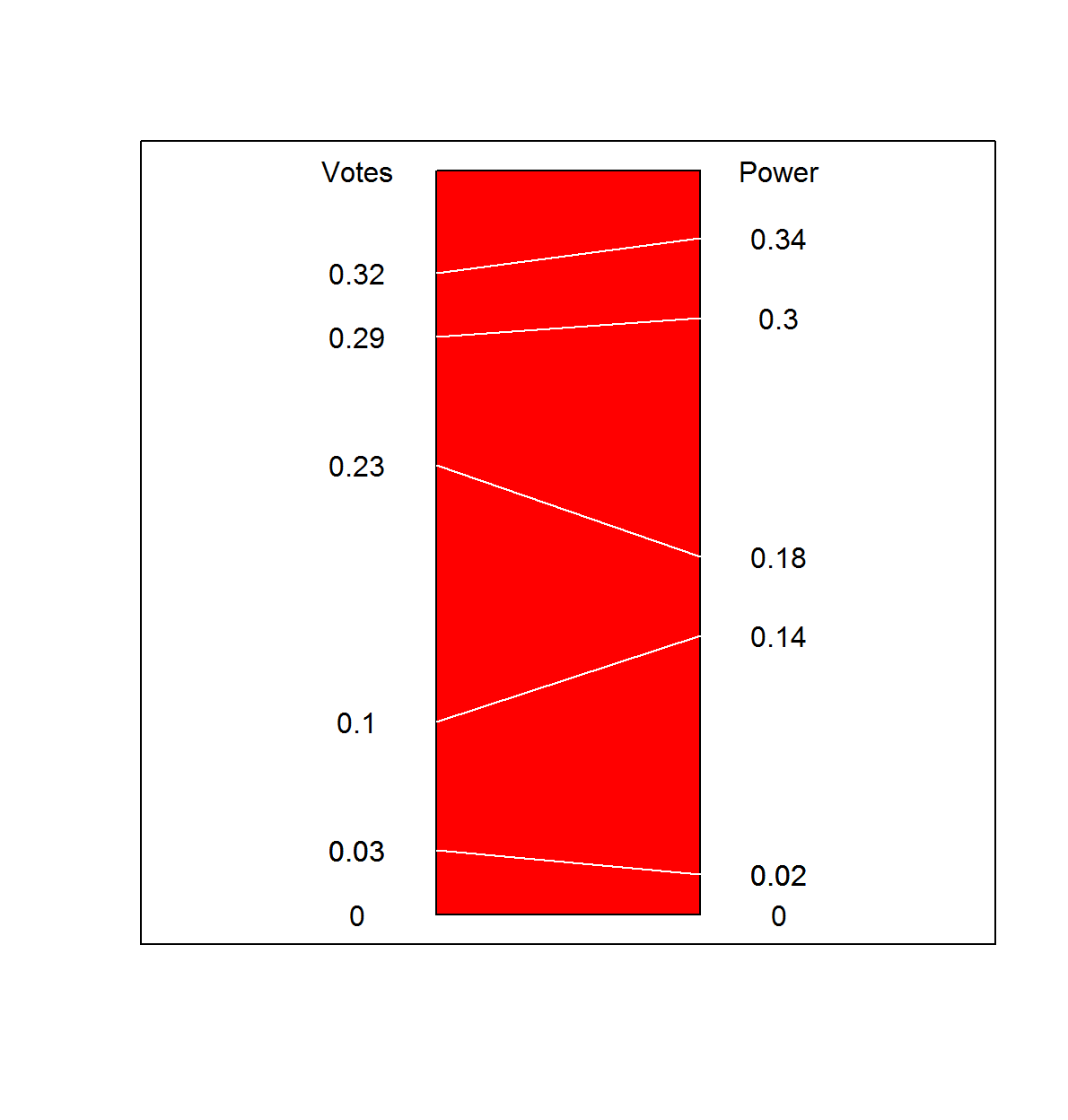Plott (#14): Illustrating the absence of stable points

``````ideals <- matrix(c(0, 0, .5, 1, 1, 0), 2)
gt_majority_phase(ideals)``````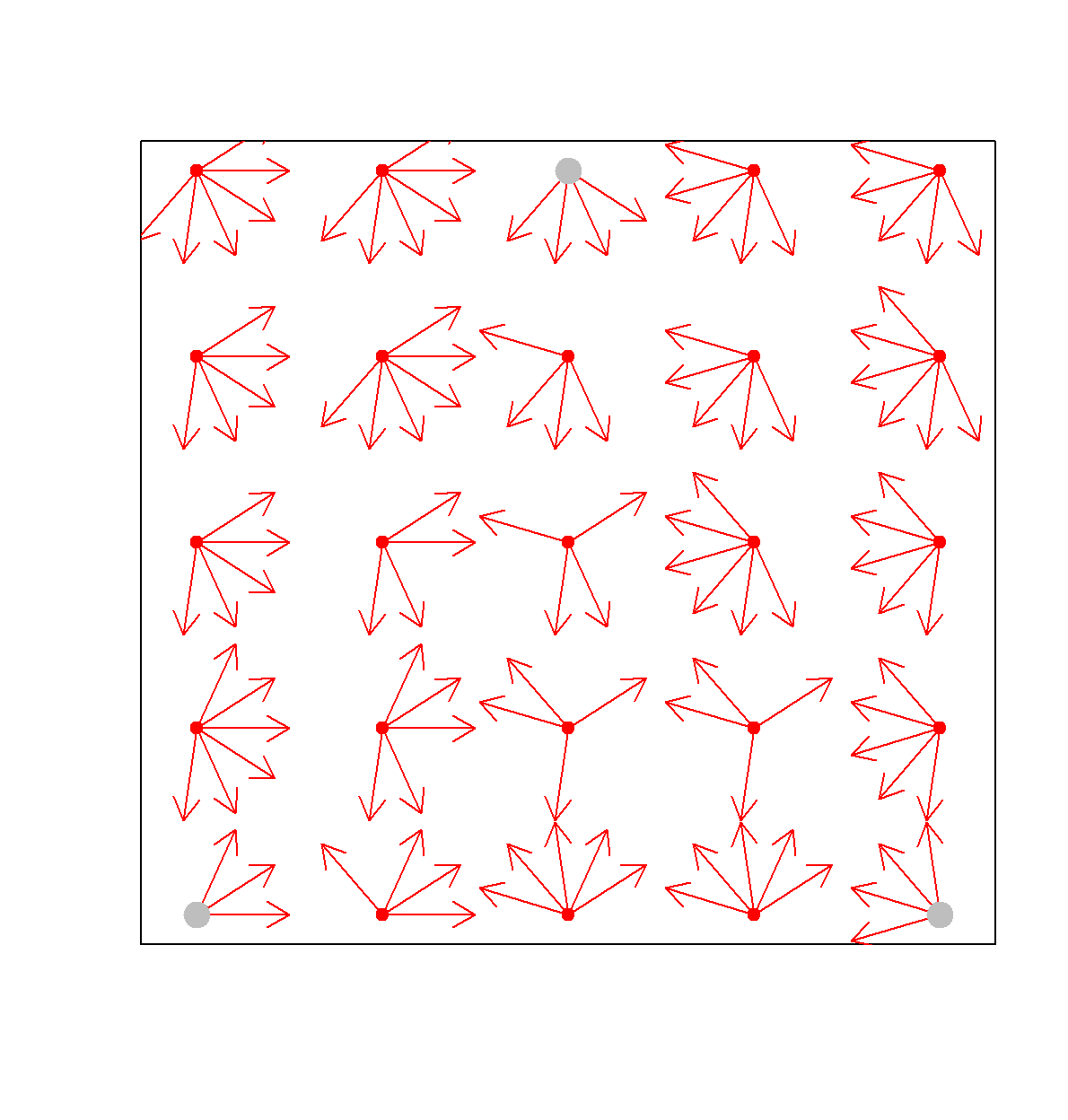``````ideals <- matrix(c(.2, .2, .5, .9, 1, 0), 2)
gt_majority_phase(ideals, raylengths=c(.5, .2))``````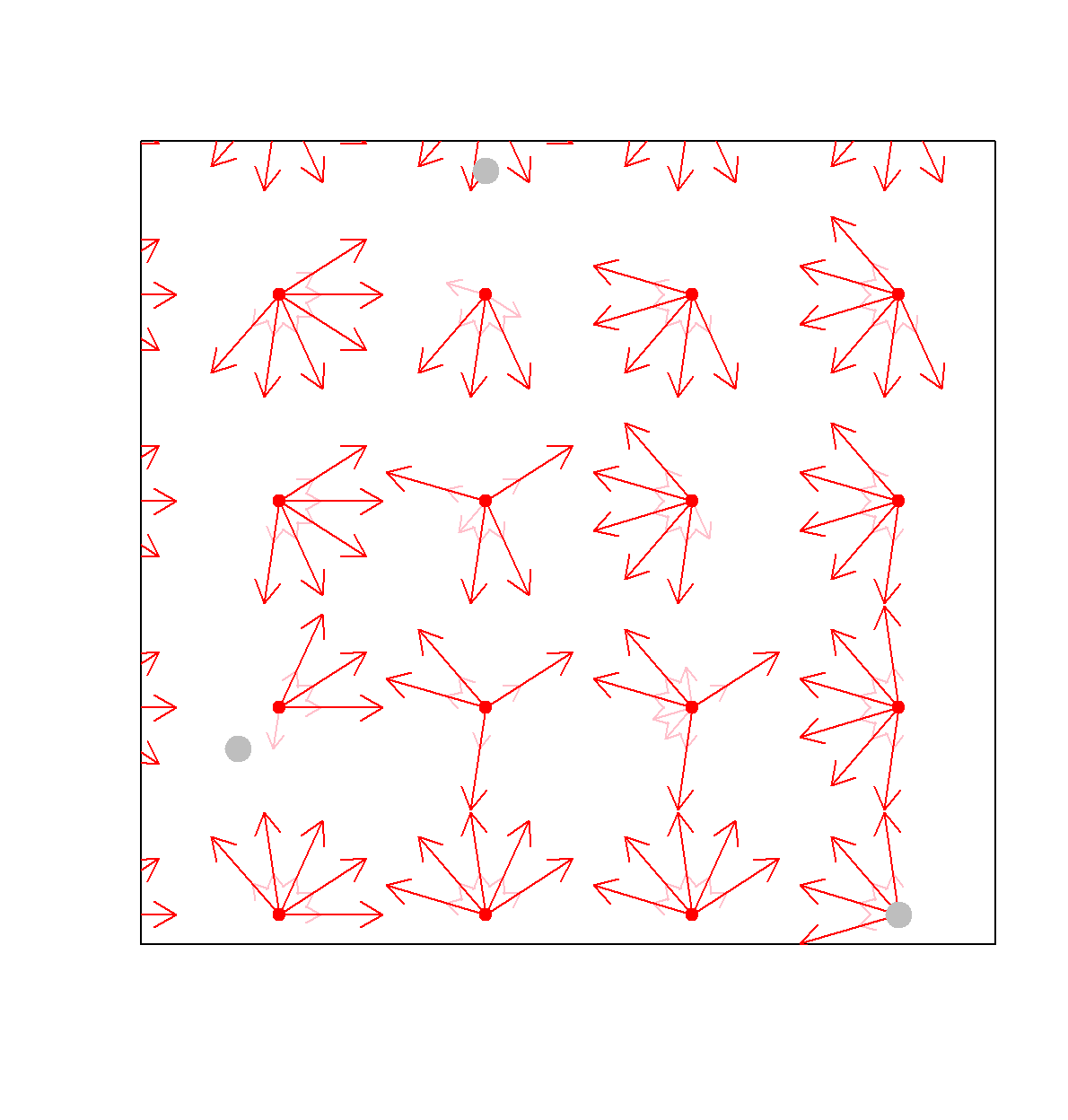Cycles (#15): Majority rule cycling

``gt_cycles(n_voters = 3, n_motions = 25)``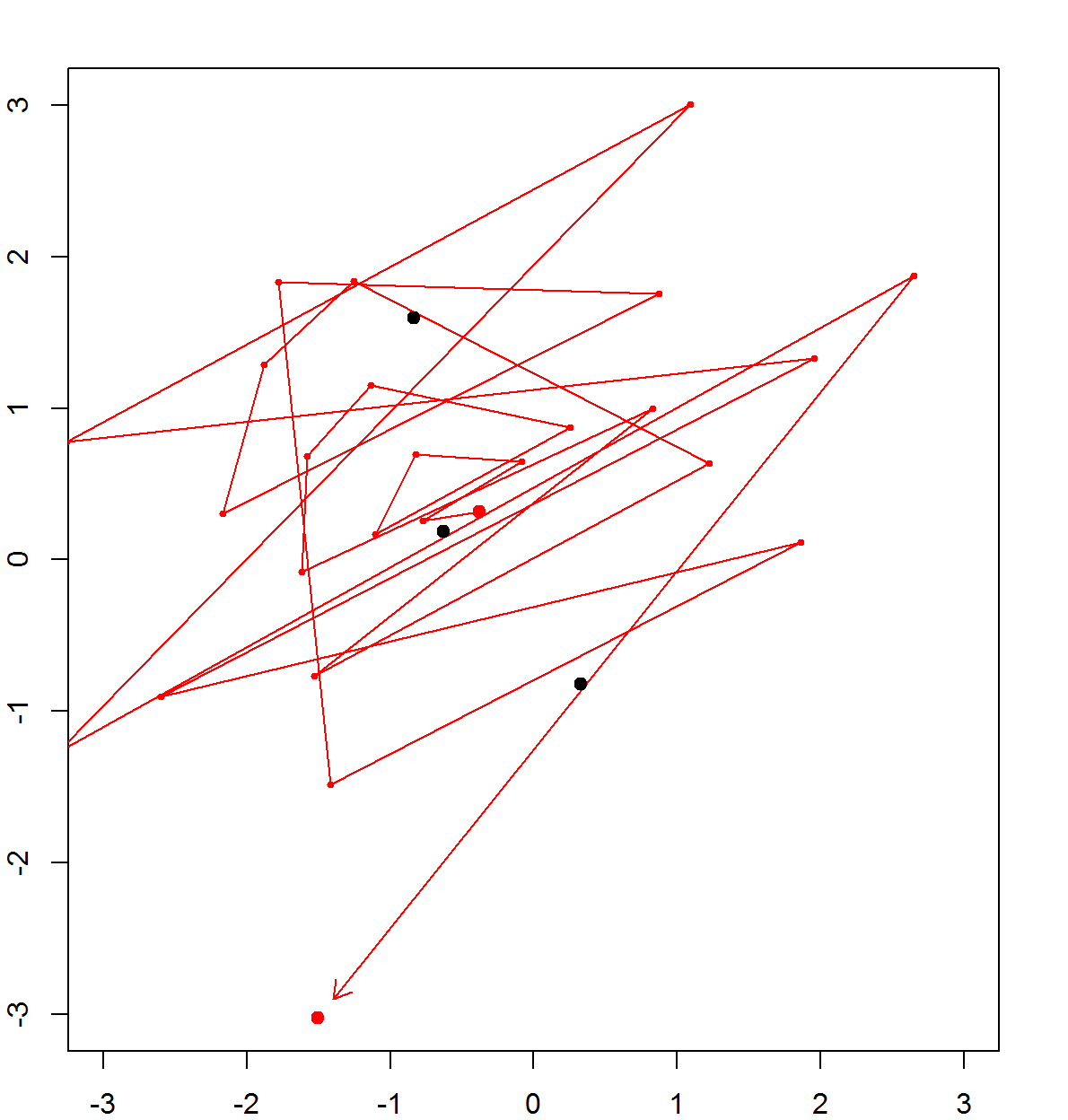Legislative bargaining (#18): Returns equilibrium returns given recognition probabilities

``````probabilities <- c(.8,.2,0)
gt_leg_barg(probabilities)``````
``##  "Anything goes: one of multiple equilibria selected"``
``##  "allocations: 0.796, 0.199, 0.005"``

Cascades (#22): Where you end up after 50,000 random orderings

``````signals <- c(1,1,1,1,1,1,0,0,0)
mean(D[9,])``````
``##  0.89284``

Nash bargaining (#27)

``gt_nbs(u1 = function(x) x^.5, u2 = function (x) 1 - x)``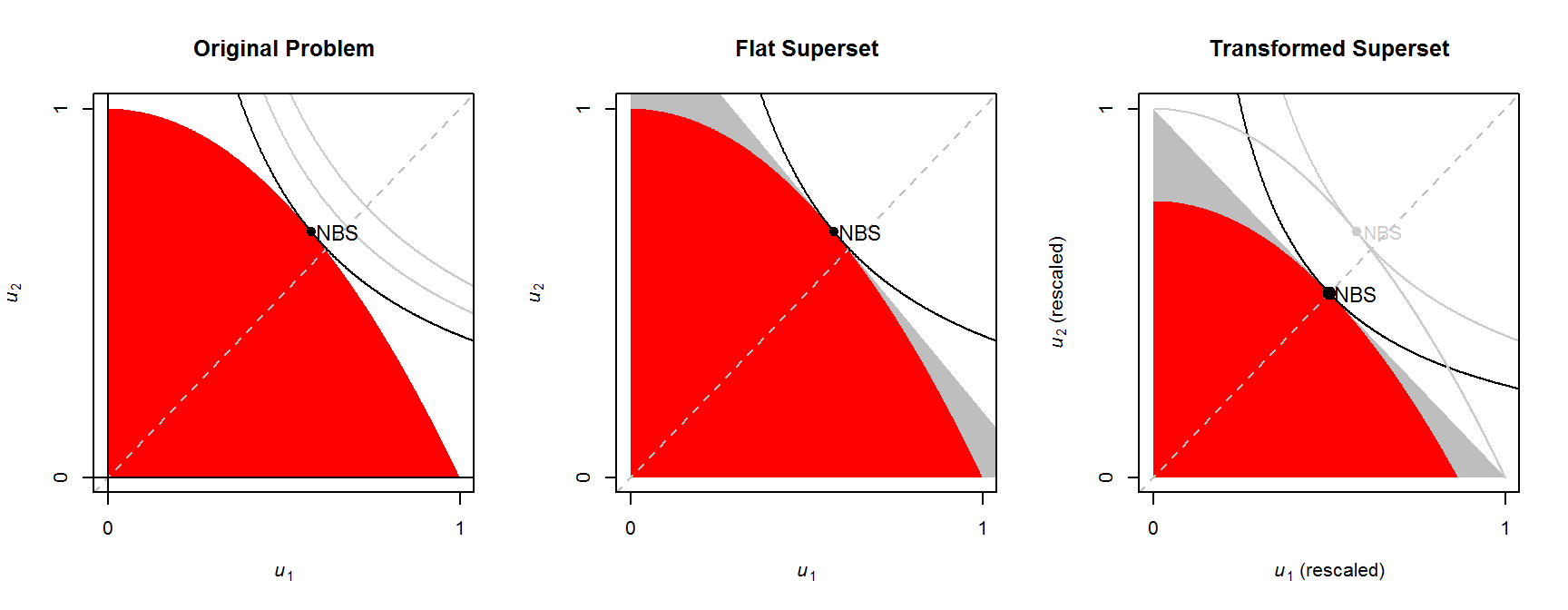Simple version with equilibrium only marked.

``gt_nbs(solution_only = T)``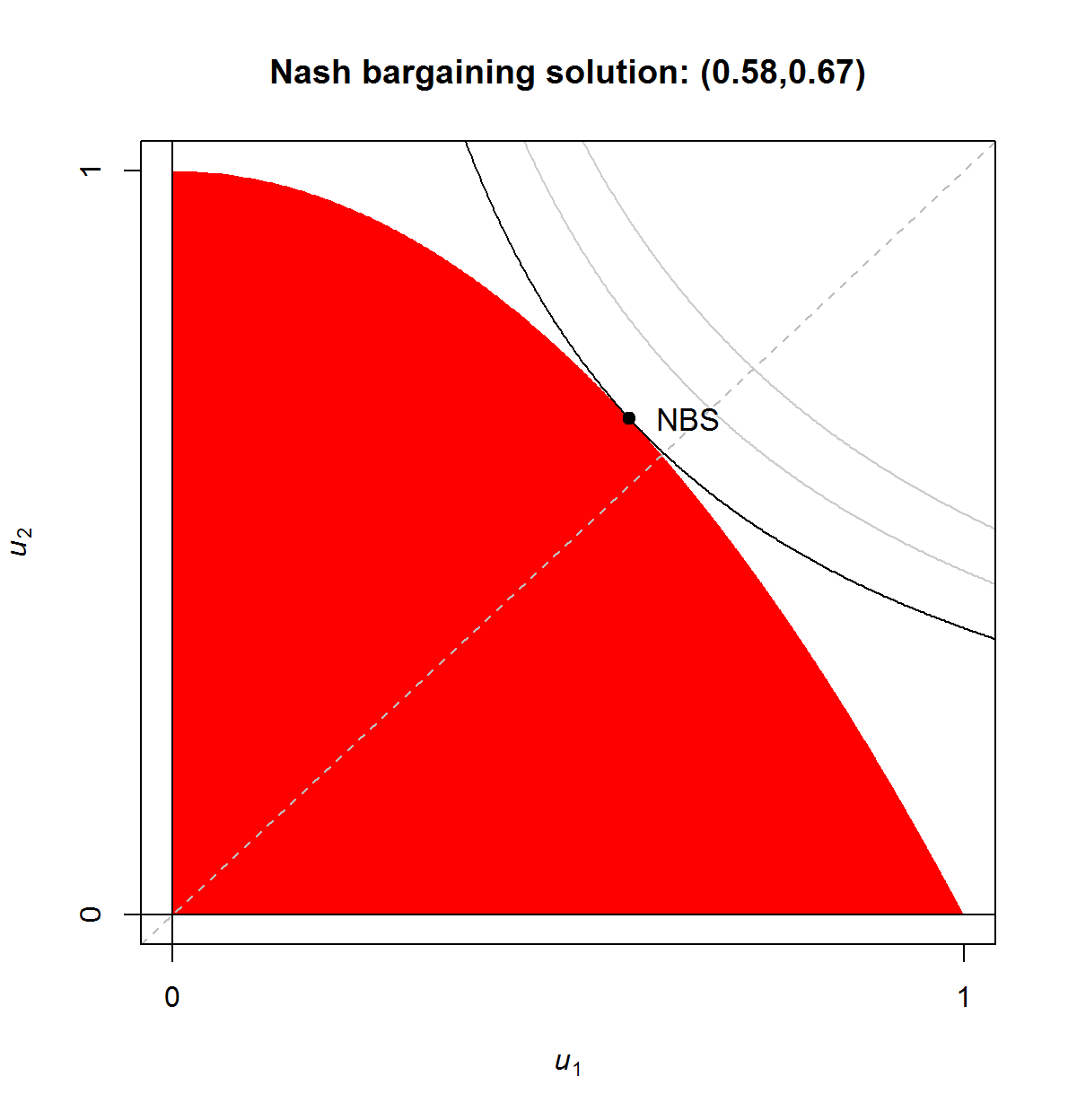Two Coase theorem illustrations (#31)

``````payoffs <- matrix(c(2,3,0,1),2)
gt_coase(payoffs, bargain = T, SQ = "minimax")``````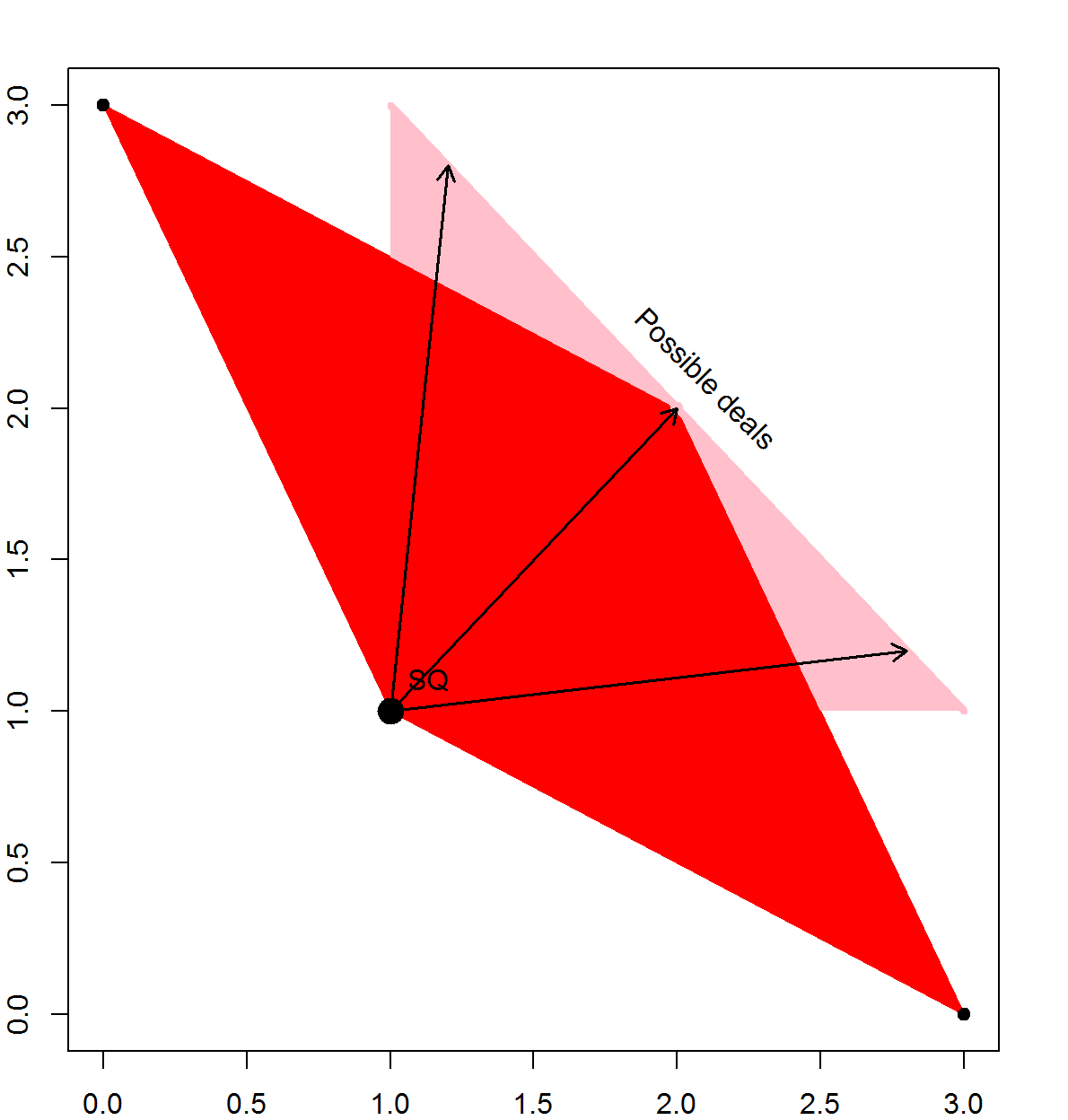``gt_coase(f=function(x) 1-x^2, bargain = T, SQ=c(0,1))``